# Analyze US Unemployment Rate Using Markov-Switching Model

This example shows how to fit a univariate Markov-switching dynamic regression model of the US unemployment rate to time series data. Also, the example shows how to simulate and forecast unemployment rate paths from the estimated model.

To fit a Markov-switching model for a univariate time series variable to data by using the `estimate` function, follow this general procedure:

1. Load and preprocess the time series data that you plan to model.

2. Examine the data to determine the key characteristics of the model, which include the number of states that the variable switches among and the structure of the state-specific submodels. Plots of the data or economic theory and principles can suggest the number of states. The structure of each submodel should be parsimonious and a good statistical description of the time series within the regime.

3. Create a partially specified Markov-switching model template for estimation by using the `msVAR` function. The model template, an `msVAR` object, specifies the structure of each state submodel and the switching mechanism among the submodels, and the parameters to fit to data.

4. Create a fully specified Markov-switching model that has the same structure as the model template. The `estimate` function uses the parameter values of this model to initialize the estimation procedure, which is the expectation-maximization algorithm.

5. Estimate the model by supplying `estimate` with the model template, initial model, and the data.

After fitting the model to data, you can pass the estimated model to the appropriate function for further analysis. For example, you can forecast the unemployment rate beyond the sample by using the `forecast` or `simulate` functions.

Load the US unemployment data set in `Data_Unemployment.mat`. The data set contains the monthly rates from 1954 through 1998.

`load Data_Unemployment`

The data set contains the 45-by-12 matrix `Data` and the numeric vector of observation years `dates`, among other variables (for more details, enter `Description` at the command line). Rows of `Data` correspond to the years 1954 through 1998 and columns correspond to the months January through December.

Econometrics Toolbox™ functions accept time series data with observations along the rows and separate variables along the columns of arrays. Reorient the rate series as one column vector containing all observations in increasing time order.

```Data = Data'; un = Data(:);```

`un` is a column vector containing the monthly unemployment rate series.

Create a `datetime` vector containing the observation times. Assume measurements are available at the beginning of each month.

```numYears = numel(dates); t = datetime(dates(1),1,1) + calmonths(0:((12*numYears)-1)); numObs = numel(t);```

### Examine Data

To determine key characteristics of the model, plot the entire unemployment rate series. Graphically distinguish periods of economic recession in the US, as determined by the National Bureau of Economic Research (NBER), by using the `recessionplot` function.

```figure plot(t,un) recessionplot ylabel("Rate (%)") title("US Unemployment Rate, 1954-1998")```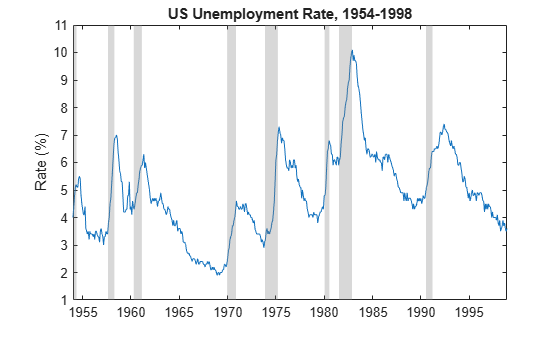Gray bands indicate periods of recession. During each recession, the rate series increases sharply with visually similar statistical characteristics, such as strong autocorrelation (perhaps close to 0.9) and volatility, among those periods. Similarly, outside periods of recession, the rate decreases with similar statistical characteristics among those periods. However, the statistical characteristics of the series within recessions and expansion periods appear different. Therefore, the plot suggests that the unemployment rate might switch between two states or submodels, and each state-specific submodel likely has an autoregressive (AR) component.

### Create Model Template for Estimation

An `msVAR` object is a variable that specifies a Markov-switching model; the `msVAR` function creates an `msVAR` object. For a univariate system, the function requires the following two inputs, which specify the key statistical characteristics of the model:

• A vector of `arima` objects, where element $\mathit{j}$ specifies the ARX($\mathit{p}$) submodel in regime $\mathit{j}$. Create each submodel by using the `arima` function. An analysis of the series within each regime, or economic theory, can suggest structures for the submodels, such as which AR lags to include. Note, however, complex model descriptions, such as those with many parameters, are a challenge for the optimization routine to fit to limited data and can result in high standard errors. A best practice is to specify parsimonious models that provide a good statistical description of the response series within the respective regimes. Also, simpler models tend to generalize better than complex models.

• A `dtmc` object, as created by the `dtmc` function, that represents the discrete-time Markov chain that describes the switching mechanism among the regimes.

Markov-switching model estimation requires the following two types of `msVAR` model objects:

• A partially specified object that serves as a template explicitly specifying the model structure and indicating which parameters to estimate. Although you must specify all the non-estimable, structural parameters, such as lags in the AR polynomial, estimable parameters, such as lag coefficients and transition probabilities, can be unknown (`NaN`-valued) or specified. `estimate` fits `NaN`-valued parameters to the data and fixes all known parameters to their specified values.

• A fully specified object, with the same model structure as the template, that specifies the initial values of all parameters for the optimization algorithm.

To show how to use the `msVAR` framework, this example sets each submodel to an AR(1) model. However, you can base your choice on submodel structures by following this procedure:

1. Fit a set of models with varying, but not overly complex, submodel structures.

2. Choose the model that performs the best. Performance measures can include in-sample fit statistics, such as AIC, or forecast MSE from a holdout sample.

Specify the structure of each state submodel by creating a vector of AR(1) model templates.

```statenames = ["Expansion" "Recession"]; mdl1 = arima(Constant=NaN,AR=NaN,Description=statenames(1)); mdl2 = arima(Constant=NaN,AR=NaN,Description=statenames(2)); submdl = [mdl1 mdl2]; mdl1```
```mdl1 = arima with properties: Description: "Expansion" Distribution: Name = "Gaussian" P: 1 D: 0 Q: 0 Constant: NaN AR: {NaN} at lag  SAR: {} MA: {} SMA: {} Seasonality: 0 Beta: [1×0] Variance: NaN ARIMA(1,0,0) Model (Gaussian Distribution) ```

`mdl1` and `mdl2` are partially specified `arima` objects representing AR(1) models containing a constant. The `NaN`-valued properties indicate which parameters to estimate. In this case and for state $\mathit{j}$, the parameters are the constant ${\mathit{c}}_{\mathit{j}}$, first AR lag ${\varphi }_{\mathit{j}}$, and innovation variance ${\sigma }_{\mathit{j}}^{2}$.

Create a two-state discrete-time Markov chain. Specify an unknown transition matrix.

`mc = dtmc([NaN NaN; NaN NaN],StateNames=statenames)`
```mc = dtmc with properties: P: [2x2 double] StateNames: ["Expansion" "Recession"] NumStates: 2 ```

`mc` is a partially specified `dtmc` object representing the switching mechanism. Because the transition matrix is composed of `NaN` values, it is prepared for estimation.

Create a Markov-switching model template for estimation by supplying the partially specified submodel vector and switching mechanism to `msVAR`. Specify the state names.

`Mdl = msVAR(mc,submdl) `
```Mdl = msVAR with properties: NumStates: 2 NumSeries: 1 StateNames: ["Expansion" "Recession"] SeriesNames: "1" Switch: [1x1 dtmc] Submodels: [2x1 varm] ```

`Mdl` is a partially specified `msVAR` object specifying the Markov-switching model structure for estimation. Ten parameters are unknown, or correspond to `NaN`-valued properties, so `estimate` estimates them.

### Create Model Containing Initial Values

The `estimate` function uses the expectation-maximization (EM) algorithm to optimize the data likelihood. The EM algorithm requires initial parameter values. `estimate` uses the parameter values of an input, fully specified Markov-switching model as the required initial values. Specified initial values do not need to be evidence based, but it is good practice to experiment with several sets of initial values to determine whether the algorithm is sensitive to them.

Base the starting values on visual inspection of the plot:

• Because the plot suggests high positive autocorrelation in both states, set the initial first AR lag to 0.9.

• Choose levels (unconditional means) of the rate as 4, for expansion, and 8, for recession.

Obtain the initial value for the constants by using these choices and the formula for the unconditional mean of a stationary AR(1) model, which is $\mu =\frac{\mathit{c}}{1-\varphi }$.

```phi0 = 0.9; muexp = 4; murec = 8; c0exp = muexp*(1 - phi0); c0rec = murec*(1 - phi0);```

Create a Markov-switching model of containing initial values for estimation. Arbitrarily set each state submodel variance to 1 and specify that transitions between states are uniformly distributed.

```sigma20= 1; mdl10 = arima(Constant=c0exp,AR=phi0,Variance=sigma20); mdl20 = arima(Constant=c0rec,AR=phi0,Variance=sigma20); P0 = 0.5*ones(2); mc0 = dtmc(P0,StateNames=statenames); Mdl0 = msVAR(mc0,[mdl10 mdl20]);```

`Mdl0` is a fully specified `msVAR` object containing the initial values for estimation.

### Estimate Model

Fit the Markov-switching model to the entire unemployment rate series. Monitor convergence of the algorithm by plotting the log-likelihood for each iteration.

`EstMdl = estimate(Mdl,Mdl0,un,IterationPlot=true);`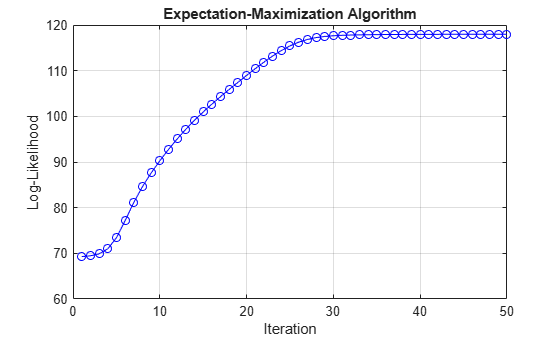The monotonically increasing log-likelihood is indicative of the behavior of the EM algorithm. The algorithm terminates after about 50 iterations, when a consecutive change is below the tolerance, as specified by the `Tolerance` name-value argument.

`EstMdl` is a fully specified `msVAR` object representing the estimated Markov-switching model. The submodels are of the same structure as those in the model template, but all parameter values are specified.

Display an estimation summary.

`summarize(EstMdl)`
```Description 1-Dimensional msVAR Model with 2 Submodels Switch Estimated Transition Matrix: 0.978 0.022 0.065 0.935 Fit Effective Sample Size: 539 Number of Estimated Parameters: 4 Number of Constrained Parameters: 0 LogLikelihood: 117.978 AIC: -227.955 BIC: -210.796 Submodels Estimate StandardError TStatistic PValue ________ _____________ __________ __________ State 1 Constant(1) 0.09672 0.021617 4.4744 7.6639e-06 State 1 AR{1}(1,1) 0.97007 0.0044692 217.06 0 State 2 Constant(1) 0.10835 0.046026 2.354 0.018572 State 2 AR{1}(1,1) 0.99926 0.0079809 125.21 0 ```

A standard statistical summary prints to the command window, including parameter estimates and their standard errors and inferences, and model fit statistics.

Plot the estimated switching mechanism.

```figure graphplot(EstMdl.Switch,ColorEdges=true,LabelEdges=true)```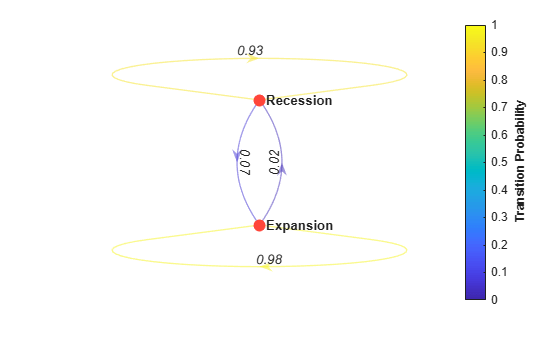The estimated transition matrix `Switch` suggests that the states are sticky, meaning they have a high probability of persisting into the next time step. This characteristic is apparent in the time series plot.

The estimated Markov-switching model is

`${y}_{t}=\left\{\begin{array}{c}0.100+0.970{y}_{t-1}+{\epsilon }_{1,t};\phantom{\rule{0.2777777777777778em}{0ex}}{s}_{t}=1\\ 0.108+0.999{y}_{t-1}+{\epsilon }_{2,t};\phantom{\rule{0.2777777777777778em}{0ex}}{s}_{t}=2\end{array}.$`

All estimates are significant at 5% level of significance.

Plot the estimated switching mechanism,.

### Forecast Unemployment Rate

A fully specified `msVAR` object represents a known Markov-switching model, which you can pass to several functions for further analysis. For example, the `msVAR` framework supports both optimal and Monte-Carlo-based point forecasting from the `forecast` function. You can also generate random response paths from a model by using the `simulate` function.

#### Forecast Using `forecast`

Forecast the estimated model 120 months beyond the in-sample measurements. Compute optimal point forecasts.

```numPeriods = 120; unOPF = forecast(EstMdl,un,numPeriods);```

`unOPF` is a 120-by-1 vector, in which `unOPF(``j``)` is the `j`-period-ahead optimal point forecast of the unemployment rate.

Compute Monte-Carlo-based forecasts by calling `forecast` again and returning the Monte-Carlo-based forecast variance (the second output argument). Base the forecasts on 1000 simulated paths.

```rng(1) % For reproducibility numPaths = 1000; [unMCF,estVar] = forecast(EstMdl,un,numPeriods, ... NumPaths=numPaths);```

`unMCF` and `estVar` are 120-by-1 vectors, where `unMCF(``j``)` and `estVar(``j``)` are the mean and variance, respectively, of the simulated unemployment rate paths at period `j`.

Plot the observed series with the optimal and Monte Carlo forecasts. Compute and plot point-wise 95% Wald confidence intervals for the forecasts.

```hrzn = t(end) + calmonths(1:numPeriods); MCFCI1 = unMCF + 1.96*[-estVar estVar]; figure hold on h1 = plot(t((end-300):end),un((end-300):end),"b"); h2 = plot(hrzn,unOPF,"r"); h3 = plot(hrzn,unMCF,"m"); h4 = plot(hrzn,MCFCI1,"--m"); hold off title("Forecast Unemployment Rate") legend([h1 h2 h3 h4(1)], ... ["Observed" "Optimal forecast" "MC forecast" "95% confidence interval"], ... Location="best")```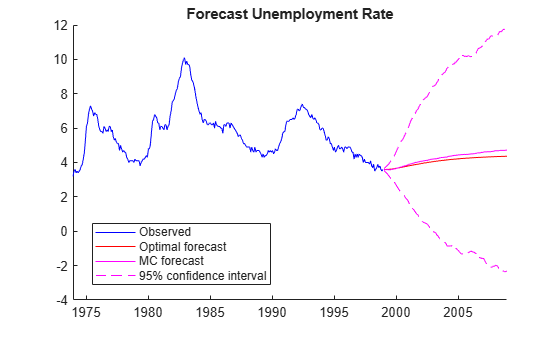The plot suggests that the uncertainty on the unemployment rate far from the in-sample data is high. In fact, the intervals are wide enough to include impossible values of an unemployment rate.

#### Forecast Using simulate

To customize a Monte Carlo study, use `simulate` to generate random response paths from a model.

To see how Markov-switching model simulation works, simulate one path from the estimated model, and then plot the path with the data.

```rng(1) unsim = simulate(EstMdl,numObs); figure plot(t,un,'b',t,unsim,'r') legend("Observed","Simulated") title("Simulated Unemployment Rate")```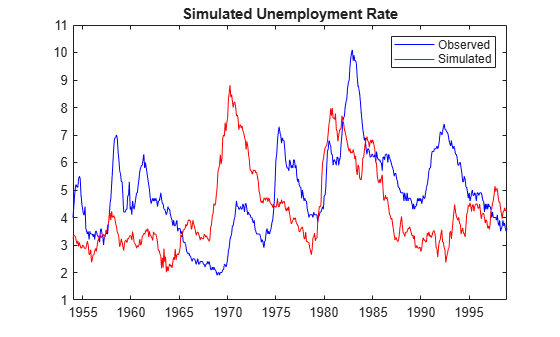`unsim` is a `numObs`-by-1 vector containing a random path simulated from `EstMdl`. The simulated path shows short clusters of sharp increases and longer clusters of slower decreases, as demonstrated by the observed series.

Forecast the estimated Markov-switching model beyond the in-sample measurements by simulating 1000 paths, computing the time-point-wise simulation means and 95% percentile intervals. Supply the in-sample data as a presample. Plot the data, the first simulated path, and the Monte Carlo forecasts and inferences.

```UNMCSim = simulate(EstMdl,numPeriods,NumPaths=numPaths,Y0=un); unmcmean = mean(UNMCSim,2); unmcint = quantile(UNMCSim,[0.025 0.975],2); figure h1 = plot(t(end-300:end),un(end-300:end),"k"); hold on h2 = plot(hrzn,UNMCSim(:,1),"r"); h3 = plot(hrzn,unmcmean,"m"); h4 = plot(hrzn,unmcint,"--m"); h5 =gca; patch([hrzn(1) h5.XLim(2) h5.XLim(2) hrzn(1)],[h5.YLim([1 1]) h5.YLim([2 2])], ... [0.75 0.75 0.75],FaceAlpha=0.2) hold off legend([h1 h2 h3 h4(1)], ... ["Observed" "Simulated path" "MC forecast" "95% confidence intervals"], ... Location="best") title("Monte Carlo Forecasted Unemployment Rate")```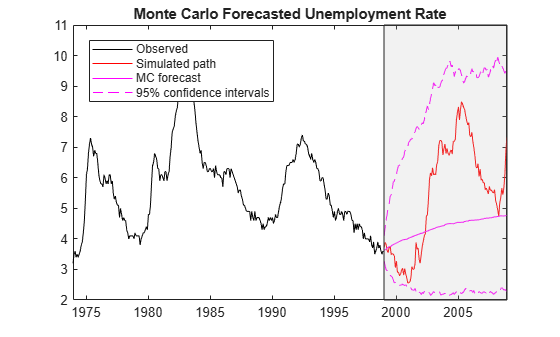`UNMCSim` is a 120-by-1000 matrix, where rows correspond to periods in the forecast horizon and columns are separate, independently simulated response paths.

Like the Wald-based confidence intervals, the percentile intervals of forecasts far from the in-sample data are wide, but the values contained in them are possible.